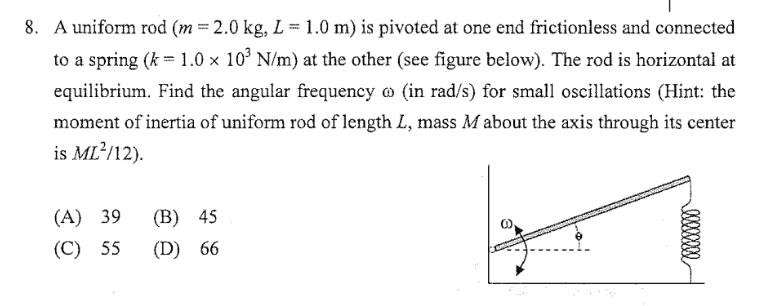# Vertical spring with a rod

## Homework Statement

Question 8E=(kx^2)/2
E=(Iw^2)/2
I=(ml^2)/3

## The Attempt at a Solution

I don't know how to start to solve the problem, because I don't really know which part should I refer to.
First I use the energy.
So the energy stored in the spring will converted to the rotational kinetic energy for the rod.
But I fail to find the x (distance of the spring compressed) Thanks

gneill
Mentor
The problem statement mentions "small oscillations", so the displacement and hence displacement angle can be taken to be small. So, keep in mind that you might be able to use small angle approximations should the situation arise.

It seems that you're to write a differential equation for the motion and find the natural frequency. For an example of a similar type of problem why don't you look into the derivation of the motion of a simple pendulum where the small angle assumption is also made.

How I derive that it is a pendulum, is it simply because the spring will bring the rod to the equilibrium when the spring is compressed or stretched?
And why it is not the case of physical pendulum?

gneill
Mentor
How I derive that it is a pendulum, is it simply because the spring will bring the rod to the equilibrium when the spring is compressed or stretched?
And why it is not the case of physical pendulum?
I didn't say that this case is a pendulum, but it's behavior is similar. For the simple or physical pendulum the restoring force is provided by gravity. In this case the restoring force is provided by the spring (so Hook's Law applies).

OK . So the horizontal position is the equilibrium, which the spring already compressed to a certain extend (change in l, and l is the length of the unstretched spring). And the force should be equal to mg to balance the weight of the rod. When the rod is oscillating, moving up a certain distance x, the net force(restoring force) will be k(change in l-x)-mg=-kx (don't know if i messed up, because I cannot get the negative sign when x>change in L) (It is a bit more complicated when it is a vertical one, instead of horizontal)

and w=sqrt(mgd/I) and I=ML/3 d is the L, length of the rod ?

gneill
Mentor
I think you can get away with ignoring the effect of gravity on the system for small oscillations, since you are essentially looking for the natural frequency of the oscillation and gravity doesn't supply a restoring force so much as an initial and constant "offset" (someone who knows better can feel free to correct me here!).

If you imagine that the image is looking down on a frictionless surface which the rod moves upon, and the relaxed position of the spring has the rod perpendicular to the wall it's pivoted from then I believe that you can find the required natural frequency from that situation. Then you're just dealing with the Δx of the spring about its natural length.

You need to look at the angular motion of the rod with torque produced by the action of the spring.

the torque produced by the action of the spring with the kΔx should be LkΔx. And Δx should be equal to Lsin(the angle), at the same time with small anglur displacement, sin(the angle)=the angle. With the torque equal Ia, w=sqrt(kL^2/I)?

gneill
Mentor
That looks good to me, although it would be good to see your derivation steps for ω.